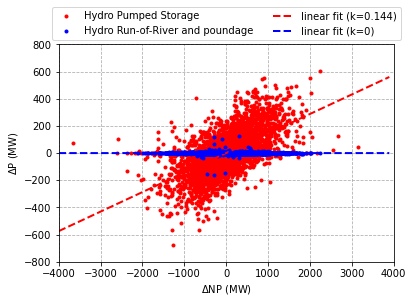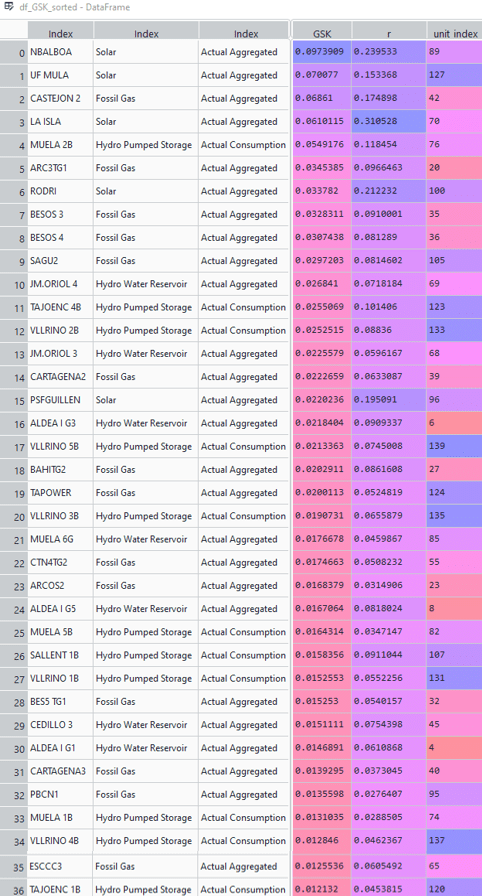# An approach to determine generation shift keys (GSKs) for defined market areas

Generation shift keys (GSKs) describe how a change of the net position of a market area affects the power outputs of the generation units within the area. In other words, the GSK describes how the change of the net position is mapped to the different generation units.

Transmission system operators (TSOs) use GSKs for various studies, e.g. load-flow studies for given market conditions. GSKs are also used in the flow-based market coupling where the cross-border capacities are determined.

Given the importance of GSKs, I have developed a straightforward approach to determine the GSKs for a market area based on data available on the ENTSO-E transparency platform (query data from the ENTSO-E transparency platform with Python). Needed are the market area’s net position and the output of the individual generation units.

## GSK – a refresher

As mentioned above, the GSK describes how to map the change of the net position to the different generation units. The formula below shows the definition of the GSK for one unit.

• GSKi … GSK of generation unit i
• ΔPi … output change of generation unit i
• ΔNP … net position change of the market area

The sum of GSKs of all generating units (1…N) must be equal to one as shown below.

## Approach to determine the GSK

In the following, I describe the approach to determine the GSK by using available data from the ENTSO-E transparency platform.

### 1) Query data

The first step is to query the data from the ENTSO-E transparency platform. The following data of a specific market area is needed:

• generation per production type
• generation per generation unit

To do that easily, the download includes a predefined Python script to query the needed data. In the script, you can easily define the timeframe and country for which you want to get the data as shown in the screenshot below. You can also define the path to where to save the data.Definition of the timeframe and country for the data query in the Python script. Additionally, you can define the path to where to save the data.

### 2) Calculate net position

The next step is to calculate the net position (NP) of the market area as shown in the formula below. The net position is defined as the aggregated generation minus load (including losses) of the market area. This means export for a positive net position and import for a negative one.

In this case, the total generation is the sum of the actual aggregated generation from each type (biomass, fossil gas, hydro, etc.) minus the actual consumption of those types. The total load including losses is directly available from the query.

### 3) Determine GSK

To identify the impact of a net position change on the output of the generation units, I assume that a change in the net position leads to a linear change of the output of the generation units. The equation below shows the linear model for one generation unit.

The plot below shows two representative examples of the relation between NP change and change of the output of the generation unit. The selected examples show a “Hydro Pumped Storage” (red) and a “Hydro Run-of-River and poundage” (blue) unit. The change of the output of the “Hydro Pumped Storage” unit is clearly connected to the change of the NP whereas the output of the “Hydro Run-of-River and poundage” unit does not show any connection to the NP change.Example for a high and low impact of the NP change on the generation unit’s output. Red: Hydro Pumped Storage, Blue: Hydro Run-of-river and poundage

The slope (k) of the two linear fits can be interpreted as the sensitivity of the generation unit’s power output to the NP change (refer to the equation above).

The next step is to calculate the sensitivity (slope) for all generating units. After that, the sensitivities can be used to calculate the GSK (which must sum up to one) by normalizing them to the sum of sensitivities as shown below.

• GSK … generation shift key for the whole market area
• k1…kN … sensitivities of all generation units

Important: Only non-zero values of the power output are used to calculate the sensitivities. Hours with zero generation(where generation unit is not producing) are removed. Sensitivities that are smaller than zero are not considered when calculating the GSK.

## Results of the GSK approach for different market areas

In this section, I present the results from the GSK approach for different market areas. The settings of the analyses are elaborated in the list and screenshot from the Python script below:

• Analysis of three market areas: Austria (AT), Germany (DE – all four areas), and Spain (ES)
• Data from January – December 2020 (entirely taken from the ENTSO-E transparency platform)
• Only generation units that operated a minimum of 5 % of the year are considered (parameter t_op_lim = 0.05 )
• GSK threshold of 1 % – smaller GSKs are removed and re-distributed to the remaining ones (parameter GSK_thresh = 0.01 )
• All months, days of the week, and hours of the day are considered (parameters sel_months, sel_daysofweek, and sel_hours as shown in the screenshot below)

### Austria

The GSK assessment for the Austrian market area gives some interesting insights. The seven highest GSKs are all related to “Hydro Pumped Storage” and “Hydro Water Reservoir”. The top three alone account for almost 50 % of the share. The rest of the GSKs is composed of “Fossil Gas” and two more “Hydro Pumped Storage” units.

As expected, “Hydro Run-of-river and poundage” units are not included in the GSK as they usually operate with constant power and, thus, are not sensitive to changes in the net position. Those units have a GSK equal to zero as shown in the plot below.

Below you can see the pandas DataFrame showing the numerical results related to the plot above. The unit_index of the plot relates to the unit_index variable in the DataFrame. The correlation between ΔNP and ΔP for each unit gives a general indication of whether the unit’s output is affected by NP changes.Austria: Numeric GSK values, correlation (r) between ΔNP and ΔP, and unit index (here, the screenshot shows non-zero values only; the DataFrame in Python shows all of them)

### Germany

Also, the German GSK results are very interesting. I have compiled all results into one plot as shown below. The first thing that stands out is that for TENNET one “Fossil Gas”-unit has a GSK = 1, while all the others are zero. Actually, it is also the only unit that has a positive correlation of the output change with the NP change which means that the units tend to reduce their output when the NP increases and vice versa.

The GSKs of the other German TSOs are distributed, as expected, among many generation units. We can also see that the TRANSNET has the fewest generation units and AMPRION the most. 50Hz is in between them.

Looking at the numbers below we can also see some interesting things. All the “Hydro Pumped Storage”-units in the GSK of TRANSNET are located in Austria. To be precise, in Vorarlberg which is the westernmost state of Austria. This is due to the fact that those units are assigned to the TENNET area.

As already mentioned above, the GSK assessment for TENNET showed that only one “Fossil Gas”-unit takes the full share and all other units are zero.

The GSK for 50Hz is mainly composed of “Hydro Pumped Storage” and “Fossil Brown coal/Lignite”-units. There are also two “Fossil Hard coal” units included.

The GSK for AMPRION is almost entirely composed of fossil-fueled units, such as “Fossil Hard coal”, “Fossil Brown coal/Lignite” and “Fossil Gas”. There is only one “Hydro Pumped Storage”-unit included. This also shows the high dependency of Germany on fossil fuels.

### Spain

The GSK assessment for the Spanish market area highlights an important consideration that should be made when assessing GSKs. When we look at the numerical results we see that the two highest GSKs are related to solar power plants. Of course, non-controllable units, such as solar or wind, need to be removed from the GSK. Here, I did not remove it in order to highlight this important point.

Looking at controllable generation units we see that the Spanish GSK is composed of gas and hydro units.Spain: Numeric GSK values, correlation (r) between ΔNP and ΔP, and unit index (here, the screenshot shows non-zero values only; the DataFrame in Python shows all of them)

• Non-controllable generation units, such as wind and PV must be removed from the GSK.
• The selection of the considered units must be made manually and according to the TSO’s operational experience.
• The correalation (r) between the unit’s output change and the NP change gives a good initial guess of units that are sensitive to a change of the NP

If this was interesting, you might also like the following: Load model identification in electric power systems: a linear least-squares approach

More insights to follow in the next article.

Michael